# Hardest colleges to get into in Minnesota

Top 10 colleges in Minnesota with the lowest acceptance rates
What are the hardest colleges to get into in Minnesota? We've got you covered. We've compiled a national college database and have created a list of the hardest universities to get into in Minnesota below. These are the hardest 4 year colleges to get into in Minnesota, and sometimes some of the hardest colleges to get into in the US. You could even say these are the best colleges in Minnesota. We also include each college's average SAT and ACT scores so that you can see where you're most competitive. Read on to find out more.

## Carleton College acceptance rate

Carleton College acceptance rate is 19%.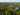The average SAT score for Carleton College is 1450.

• SAT composite: 1450
• SAT math: 740

The average ACT score for Carleton College is 33.

## Macalester College acceptance rate

Macalester College acceptance rate is 41.2%.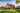The average SAT score for Macalester College is 1411.

• SAT composite: 1411
• SAT math: 720

The average ACT score for Macalester College is 30.

## Augsburg College acceptance rate

Augsburg College acceptance rate is 45%.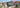The average SAT score for Augsburg College is 1130.

• SAT composite: 1130
• SAT math: 540

The average ACT score for Augsburg College is 22.

## Saint Olaf College acceptance rate

Saint Olaf College acceptance rate is 49.9%.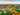The average SAT score for Saint Olaf College is 1300.

• SAT composite: 1300
• SAT math: 650

The average ACT score for Saint Olaf College is 29.

## Concordia University Saint Paul acceptance rate

Concordia University Saint Paul acceptance rate is 54.9%.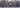The average SAT score for Concordia University Saint Paul is 1080.

• SAT composite: 1080
• SAT math: 540

The average ACT score for Concordia University Saint Paul is 21.

## Crown College acceptance rate

Crown College acceptance rate is 56.2%.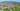The average SAT score for Crown College is 1140.

• SAT composite: 1140
• SAT math: 560

The average ACT score for Crown College is 22.

## University of Minnesota acceptance rate

University of Minnesota acceptance rate is 56.7%.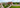The average SAT score for University of Minnesota is 1352.

• SAT composite: 1352
• SAT math: 703

The average ACT score for University of Minnesota is 28.

## Minnesota State University Moorhead acceptance rate

Minnesota State University Moorhead acceptance rate is 60.3%.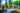The average SAT score for Minnesota State University Moorhead is 1060.

• SAT composite: 1060
• SAT math: 540

The average ACT score for Minnesota State University Moorhead is 23.

## Minnesota State University Mankato acceptance rate

Minnesota State University Mankato acceptance rate is 61%.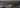The average SAT score for Minnesota State University Mankato is 1110.

• SAT composite: 1110
• SAT math: 555

The average ACT score for Minnesota State University Mankato is 22.

## Concordia College acceptance rate

Concordia College acceptance rate is 65.1%.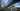The average SAT score for Concordia College is 1200.

• SAT composite: 1200
• SAT math: 580